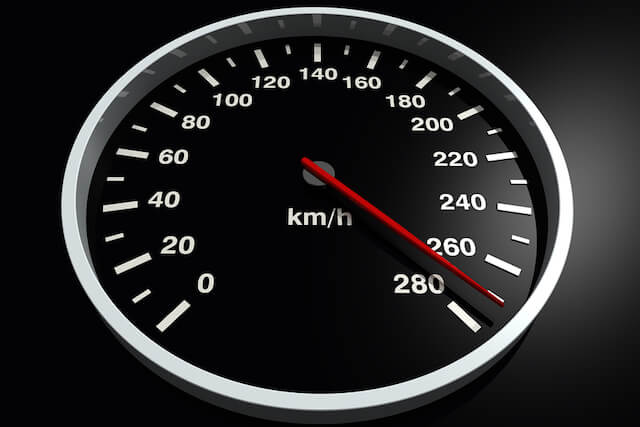To the layman, speed, velocity and acceleration may just sound like different ways to describe how fast you are going, but they actually mean very different things. The distinction between each term is essential to the study of kinematics – the study of how objects move. In this article, we will go through what each term means, discuss the differences between them, and provide some formulas to help you understand the concepts inherent to the terms.

Speed

Out of the three terms, the definition of speed in physics is the closest to the layman’s understanding of it. Speed is defined as the distance moved by an object or particle in a particular period of time. The formula is rather straightforward:

• Speed = distance travelled / time taken
• Unit: m/s or ms-1

The most common speed you will encounter is the speed of light. The speed of light in a vacuum is a constant at 299,792km/s. This makes the speed of light, or the amount of distance it travels in a fixed amount of time, a good baseline to define other terms. For example, a metre is defined as the distance travelled by light in 1/299,792,458 of a second.

Velocity

Velocity and speed are often conflated in the layman’s understanding. However, in physics, the difference between them can be night and day. If you think back to the concept of scalars and vectors, you may recall that speed is a scalar, while velocity is a vector. While speed only tells you how fast something is going, velocity represents both how fast an object is going, as well as its direction.

To define velocity, we have to understand a term called “displacement”. The displacement of an object shows the change in position relative to an initial point. Take, for example, a man walking along a straight road facing the east. Consider if he walks 20m towards the east, then turns back and walks 15m towards the west. While he has travelled 35m in total, he is only 5m from his initial starting point. Hence, his displacement is 5m.

With this in mind, here is the formula for velocity:

• Velocity = displacement / time taken
• Unit: m/s or ms-1

To better illustrate the difference between speed and velocity, consider the man in the above case again, and assume that his trip took 100s in total. His speed would be 35/100 = 0.35ms-1, while his velocity would be 5/100 = 0.05 ms-1.

An important term to remember is uniform velocity. Uniform velocity simply refers to a constant velocity. In other words, the object is travelling away from the initial point at a constant rate in a given direction. In contrast, non-uniform velocity is when velocity changes constantly. This means that the object covers equal displacement in unequal time intervals. This can occur when an object is accelerating, decelerating, or changing direction.

Acceleration

Velocity and acceleration are intertwined concepts. Acceleration is a measurement that represents the rate of change of the velocity of an object. Negative acceleration is often referred to as deceleration, but for the sake of simplicity, we will stick to the term acceleration to discuss the concept. There are two main types of acceleration: Average acceleration and instantaneous acceleration. Average acceleration is defined by the following equation:

• Average acceleration = change in velocity / time taken
• Unit: m/s2 or ms-2

This refers to the overall rate of change of velocity. If you want to find the acceleration at a specific time point, you can use a velocity-time graph. The simplest method is to draw a tangent to the graph at the time point of interest. This allows you to find the gradient at that point, which represents the rate of change of velocity at that point, i.e. the instantaneous acceleration.

Another important term is uniform acceleration. Like uniform velocity, uniform acceleration refers to a constant acceleration. In other words, velocity changes at a constant rate. An example of this is the acceleration of free fall. This refers to the uniform acceleration with which objects fall to the earth due to gravity. The acceleration of free fall near the surface of the earth is approximately 9.81 ms−2.

Speed, velocity and acceleration are all measurements that deal with how fast an object is moving, but now you know that they are describing very different things. While the main concepts are not too hard to understand, questions can get tricky once other kinematics concepts like projectile motion are introduced. If you find yourself in need of help for kinematics or any other physics concept, Best Physics Tuition has a fun and enriching physics tuition programme for O Level and A Level physics students.